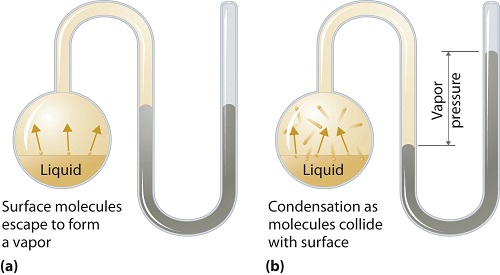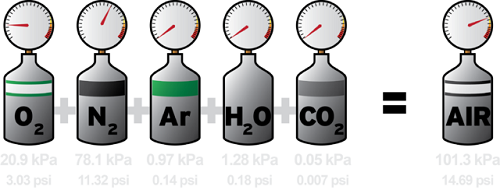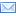#Difference Between Similar Terms and Objects

# Difference between Partial Pressure and Vapor Pressure

Partial pressure and vapor pressure are commonly used scientific terms relating to the amount of pressure exerted by system components, but their identity can be confusing to others. There is a clear distinction between these terms including their effects and identity. This article will elaborate more on the differences between these terms. It will also include some examples to demystify the applications thereof.

Let’s start by highlighting the concept of pressure before we can delve into the distinction between vapor and partial pressure. Pressure is scientifically defined as the force applied per unit area on an object or a substance. It can also be defined as the force applied by the colliding particles on each other and it is often measured using Pascal. In the case of collision of particles, the gas equation and kinetic theory of gases is used to calculate the pressure.## What is vapor pressure?

Vapor pressure can apply to liquid or solid phases. It is the pressure exerted by the vapor in its thermodynamic equilibrium on its liquid or solid state at a given temperature in a closed system when both the vapor and the liquid (solid) are in contact. This pressure arises as a result of vaporization, which is enabled by an increased heat on the solid or liquid. Thus the temperature is used as the measure of vaporization and it is directly proportional to the vapor pressure. This means, the higher the temperature is the higher the vapor pressure.

During vaporization, the air molecules escape as a result of the higher kinetic energy to the air in a closed system. Then when in equilibrium, the vapor pressure arises between the vapor and its condensed form of liquid (solid). In solutions where the intermolecular forces are weaker, the vapor pressure tends to be more, and, conversely, in solutions where the intermolecular forces are stronger, the vapor pressure is less.

The vapor pressure can also occur in ideal mixtures as explained by the Raoult’s Law. It states that the partial vapor pressure of a particular component in a liquid or solid mixture is equal to the vapor pressure of that component multiplied by its mole fraction in that mixture at a given temperature. The example below will illustrate that.

Example 1.

Given an ideal mixture of 0.5 mol. ethanol and 1.5 mol. methanol with the vapor pressure of 30KPa and 52KPa, respectively, determine the partial vapor pressure of each component.

Solution:

The total number of moles is 1.5mol + 0.5mol = 2.0mol. According to Raoult’s Law, the partial vapor pressure is equal to the vapor pressure multiplied by the molar fraction of that particular component. In this case, the Pmethanol = 1.5/2 * 52 = 39KPa and Pethanol = 0.5/2 * 30 = 7.5KPa.

When you have the partial vapor pressures of the components in the mixture, you can get the total vapor pressure by adding them together. In this regard, 7.5 + 39 gives 46.5KPa total vapor pressure of the mixture of ethanol and methanol solutions.

### Factors affecting the vapor pressure

Molecules identity

As already mentioned above, the types of molecular forces determine the amount of vapor pressure to be exerted. If the forces are stronger, then less vapor pressure emerges, and if weaker, then more vapor pressure arises. Therefore, the composition of the liquid or solid will affect the vapor pressure.

Temperature

Higher temperature leads to higher vapor pressure because it activates more kinetic energy to break the molecular forces so that the molecules can escape the liquid surface quickly. When the vapor pressure (saturated vapor pressure) equals the external pressure (atmospheric pressure) the liquid will start to boil. A lower temperature will result with low vapor pressure and it will take time for the liquid to boil.Dalton’s Law of Partial Pressures

## What is partial pressure?

The idea of partial pressure was first proposed by the renowned scientist John Dalton. It gave birth to his Law of Partial Pressures which states that the total pressure exerted by an ideal mixture of gases is equal to the sum of the partial pressures of individual gases. Say a particular container is filled with Hydrogen, Nitrogen and Oxygen gases, the total pressure, PTOTAL, will be equal to the sum of oxygen, nitrogen and hydrogen. The partial pressure of any gas in that mixture is calculated by multiplying the total pressure by the molar fraction of the individual gas.

In a nutshell, partial pressure is the pressure exerted by a particular gas in the mixture as if it were acting alone in the system. Thus you ignore other gases when determining the partial pressure of an individual gas. This theory can be verified by injecting, say, 0.6atm of O2 in a 10.0 L container at a 230K and then injecting 0.4atm N2 in an identical container of the same size at the same temperature, and then ultimately combine the gases to measure the total pressure; it will be the sum of the two gases. This clearly explains the partial pressure of an individual gas in a mixture of non-reactive gases.

### Calculating the partial pressure

To calculate the partial pressure is an absolute breeze since the Dalton’s law provides provisions for that. It will depend on the typical information provided. If, for example, the total pressure is given for a mixture of gas A and B as well as the pressure of gas A, the partial pressure of B can be calculated by using PTOTAL = PA + PB. The rest is algebraic manipulations. But in a case where only the total pressure of the mixture has been given, you can use the molar fraction of gas B to determine is partial pressure. The molar fraction, denoted by X, can be found by dividing the moles of gas B by the total moles of the gas mixture. Then to find the partial pressure, you will multiply the molar fraction, X, by the total pressure. The example below elaborates that.

Example 2.

A mixture of Nitrogen and Oxygen, with 2.5 moles and 1.85 moles, respectively, is injected in a 20.0L container with a total pressure of 4atm; calculate the partial pressure exerted by the Oxygen gas.

Solution:

The total number of moles in the mixture is 2.5 + 1.85 = 4.35 moles. So the molar fraction of Oxygen, Xo, will be 1.85 moles/4.35 moles = 0.425 moles. The partial pressure of Oxygen will be 0.425 * 4atm = 1.7atm. The partial pressure of the remaining gas can be calculated following the same approach or can be calculated by using the Oxygen gas and the total pressure as elaborated by Dalton’s law of Partial Pressures that the total pressure of non-reactive gases is equal to the sum of the partial pressures.

### Difference between vapor and partial pressure

From above explanations, it is apparent that vapor pressure and partial pressure are two distinct pressures. Vapor pressure applies on liquid and solid phases while the partial pressure applies on the gaseous phase. The vapor pressure is exerted in phase transition after sufficient heat has been added to the solution thus leading to its molecules to escape in a closed system.

The main difference between partial pressure and vapor pressure is that partial pressure is the pressure exerted by an individual gas in a mixture as if it were alone in that system, while the vapor pressure refers to the pressure exerted by the vapor in its thermodynamic equilibrium with its condensed state of liquid or solid. The table below gives a concise comparison of these pressures.

 Vapor Pressure Partial Pressure It is exerted by liquid or solid vapor on its condensed phase in equilibrium It is exerted by individual gases in a non-reactive gas mixture Well explained by Raoult’s Law Well explained by Dalton’s Law Applicable in solid and liquid phases Applicable in gaseous phases only Independent of the surface area or the volume of the system Calculated using the gases in the same volume Calculated using the mole fraction of the solute Calculated using the mole fraction of the gas

### Wrap Up!

Vapor pressure and partial pressure are two important scientific terms used in determining the effects of the forces applied by the vapor and the gases, respectively, in given closed system at particular temperatures. Their main difference is the application area with the vapor pressure applied on liquid or solid phases, whereas the partial pressure is applied on an individual gas in a mixture of ideal gases in a given volume.

The partial pressure is calculated easily by following the Dalton’s Law of Partial Pressures, whereas the vapor pressure is calculated by applying the Raoult’s Law. In any given mixture, each gas component exerts its own pressure, which is called the partial pressure independent of other gases. And when you double the moles of any component with the temperature remaining constant, you will be increasing its partial pressure. According to Clausius–Clapeyron relation, the vapor pressure increases as the temperature increases.

With the aforementioned information, you should be able to distinguish between vapor pressure and partial pressure. You should also be able to calculate them using the molar fractions and multiplying by the total pressure. We have given you typical examples to elaborate on the application of these pressures.

### Search DifferenceBetween.net :

Custom Search

Help us improve. Rate this post!Loading...Email This Post : If you like this article or our site. Please spread the word. Share it with your friends/family.

1. That was very helpful. Thank you very much!

2. thank u so much for this…..

3. Explanation was quite meaningful. Thanks for the same.

4. I think here in this sentence, where “vapor pressure” is explained, something is wrong.
“During vaporization, the air molecules escape as a result of the higher kinetic energy to the air in a closed system.”
Since we are talking about pure substances, not mixtures, no air is present in our closed system. Therefore, I think what was meant here is “During vaporization, the vaporizing molecules escape the liquid (solid) as a result of the higher kinetic energy compared to that of the vapor in a closed system.”
Or does the author talk about the air dissolved in a liquid? In that case, the text is very unclear.

Please note: comment moderation is enabled and may delay your comment. There is no need to resubmit your comment.

## References :

https://www.boundless.com/chemistry/textbooks/boundless-chemistry-textbook/gases-5/partial-pressure-54/dalton-s-law-of-partial-pressure-261-7267/

http://www.science.uwaterloo.ca/~cchieh/cact/c123/clausius.html

https://2012books.lardbucket.org/books/principles-of-general-chemistry-v1.0/s15-04-vapor-pressure.html

https://en.wikipedia.org/wiki/Dalton%27s_law

https://en.wikipedia.org/wiki/Dalton%27s_law

Articles on DifferenceBetween.net are general information, and are not intended to substitute for professional advice. The information is "AS IS", "WITH ALL FAULTS". User assumes all risk of use, damage, or injury. You agree that we have no liability for any damages.